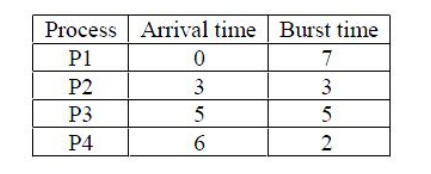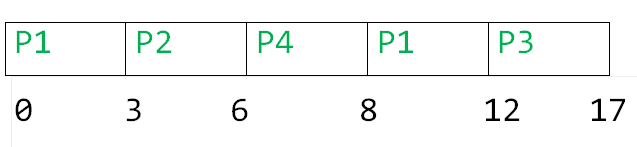Related Articles

# GATE | Sudo GATE 2020 Mock II (10 January 2019) | Question 16

• Last Updated : 07 Jan, 2020

Consider the following CPU processes with arrival times (in milliseconds) and length of CPU bursts (in milliseconds) as given below:If the pre-emptive shortest remaining time first scheduling algorithm is used to schedule the processes, then the average turn around time across all processes is _______ milliseconds.
(A) 3
(B) 7
(C) 7.25
(D) 7.50

Explanation: Gantt chart is,Turn Around Time:

```P1  = 12-0 = 12
P2 = 6-3 = 3
P3 = 17-5 = 12
P4 = 8 - 6 = 2 ```

So, average turn around time is,

`= (12+3+12+2) / 4 = 7.25`

Option (C) is correct.

Quiz of this Question

Attention reader! Don’t stop learning now.  Practice GATE exam well before the actual exam with the subject-wise and overall quizzes available in GATE Test Series Course.

Learn all GATE CS concepts with Free Live Classes on our youtube channel.

My Personal Notes arrow_drop_up